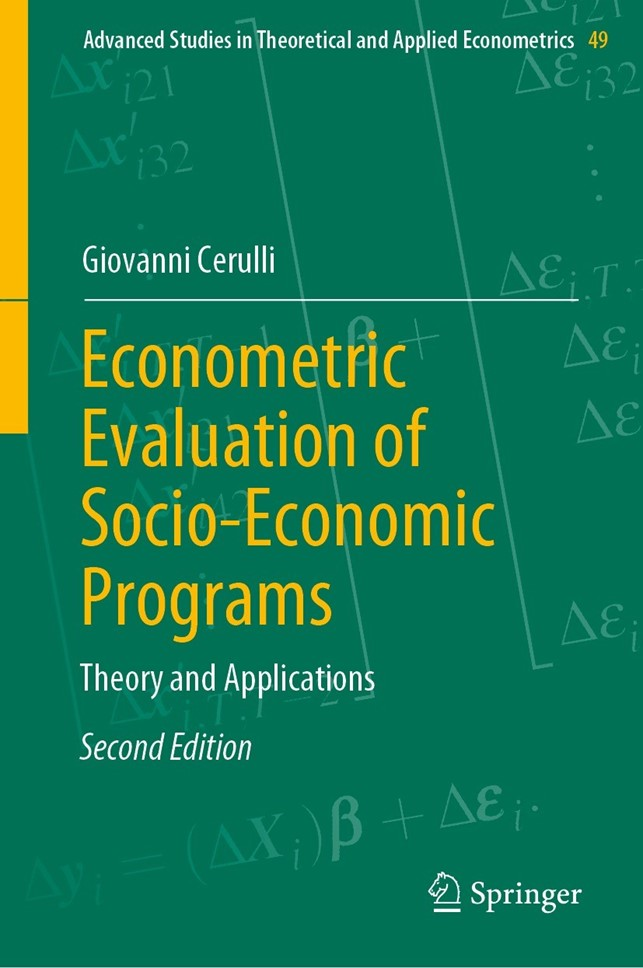# Econometric Evaluation of Socio-Economic Programs | Theory and Applications

Econometric Evaluation of Socio-Economic Programs by Giovanni provides advanced theoretical and applied tools for the implementation of modern micro-econometric techniques in evidence-based program evaluation for the social sciences. The author presents a comprehensive toolbox for designing rigorous and effective ex-post program evaluation using the statistical software package Stata. For each method, a statistical presentation is developed, followed by a practical estimation of the treatment effects. By using both real and simulated data, readers will become familiar with evaluation techniques, such as regression-adjustment, matching, difference-in-differences, instrumental-variables, regression-discontinuity-design, and synthetic control method, and are given practical guidelines for selecting and applying suitable methods for specific policy contexts.

The second revised and extended edition features two new chapters on some recent development of difference-in-differences. Specifically, chapter 5 introduces advanced difference-in-differences methods when many times are available and treatment can be either time-varying or fixed at a specific time. Chapter 6 introduces the synthetic control method, a treatment effect estimation approach suitable when only one unit is treated. Both chapters present applications using the software Stata.

AN INTRODUCTION TO THE ECONOMETRICS OF PROGRAM EVALUATION

Introduction
Statistical Setup, Notation, and Assumptions

Identification Under Random Assignment
A Bayesian Interpretation of ATE Under Randomization
Consequences of Nonrandom Assignment and Selection Bias

Selection on Observables and Selection on Unobservables

Selection on Observables (or Overt Bias) and Conditional Independence Assumption
Selection on Unobservables (or Hidden Bias)
The Overlap Assumption

Characterizing Selection Bias

Decomposing Selection Bias

The Rationale for Choosing the Variables to Control for
Partial Identification of ATEs: The Bounding Approach
A Guiding Taxonomy of the Econometric Methods for Program Evaluation
Policy Framework and the Statistical Design for Counterfactual Evaluation
Available Econometric Software
A Brief Outline of the Book

References

METHODS BASED ON SELECTION ON OBSERVABLES

Introduction

Regression-Adjustment as Unifying Approach Under Observable Selection
Linear Parametric Regression-Adjustment: The Control-Function Regression

Matching

Covariates and Propensity-Score Matching
Identification of ATEs Under Matching
Large Sample Properties of Matching Estimator(s)
Common Support
Exact Matching and the “Dimensionality Problem”
The Properties of the Propensity-Score
Quasi-exact Matching Using the Propensity-Score
Methods for Propensity-Score Matching
Inference for Matching Methods
Assessing the Reliability of CMI by Sensitivity Analysis
Assessing Overlap
Coarsened-Exact Matching

Reweighting

Reweighting and Weighted Least Squares
Reweighting on the Propensity-Score Inverse-Probability
Sample Estimation and Standard Errors for ATEs

Doubly-Robust Estimation
Implementation and Application of Matching

Covariates Matching
Propensity-Score Matching
An Example of Coarsened-Exact Matching Using cem

Implementation and Application of Reweighting

The Stata Routine treatrew
The Relation Between treatrew and Stata’s teffects ipw
An Application of the Doubly-Robust Estimator

References

METHODS BASED ON SELECTION ON UNOBSERVABLES

Introduction
Instrumental-Variables

IV Solution to Hidden Bias
IV Estimation of ATEs
IV with Observable and Unobservable Heterogeneities
Problems with IV Estimation

Selection-Model

Characterizing OLS Bias within a Selection-Model
A Technical Exposition of the Selection-Model
Selection-Model with a Binary Outcome

Difference-in-Differences

DID with Repeated Cross Sections
DID with Panel Data
DID with Matching

Implementation and Application of IV and Selection-Model

The Stata Command ivtreatreg
A Monte Carlo Experiment
An Application to Determine the Effect of Education on Fertility
Applying the Selection-Model Using etregress

Implementation and Application of DID

DID with Repeated Cross Sections
DID Application with Panel Data

References

LOCAL AVERAGE TREATMENT EFFECT AND REGRESSION-DISCONTINUITY-DESIGN

Introduction
Local Average Treatment Effect

Randomization Under Imperfect Compliance
Wald Estimator and LATE
LATE Estimation
Estimating Average Response for Compliers
Characterizing Compliers
LATE with Multiple Instruments and Multiple Treatment

Regression-Discontinuity-Design

Sharp RDD
Fuzzy RDD
The Choice of the Bandwidth and Polynomial Order
Testing RDD Reliability
A Protocol for Practical Implementation of RDD

Application and Implementation

An Application of LATE
An Application of RDD by Simulation

An application of RRD to real Data

References

DIFFERENCE-IN-DIFFERENCE WITH MANY PRE- AND POST-TREATMENT TIMES

Introduction

The TVDIFF Model

Testing the “Common Trend” Assumption
An Application of the TVDIFF Model

The TFDIFF Model

Generalization to More Than Three Times
Testing the Parallel-Trend Assumption
An Application of the TFDIFF Model

Parallel-Trend Test in the Presence of Effect Anticipation
Conclusion

References

SYNTHETIC CONTROL METHOD

Introduction
The SCM Model
SCM Inference
Application
Conclusions

ReferencesAuthor: Giovanni Cerulli
Edition: 2nd revised and extended edition
ISBN-13: 978-3-662-65945-8